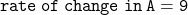## Two functions, A and B, are described as follows: Function A y = 9x + 4 Function B The rate of change is 3 and the y-intercept is 4. How muc

Question

Two functions, A and B, are described as follows: Function A y = 9x + 4 Function B The rate of change is 3 and the y-intercept is 4. How much more is the rate of change of function A than the rate of change of function B?

2
3
6
9​

in progress 0
6 months 2021-07-27T15:04:57+00:00 1 Answers 12 views 0

## Answers ( )